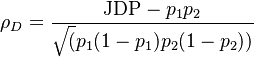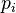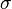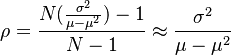# Default Correlation

## Definition

Default Correlation denotes a measure of Default Dependency between different borrowers when considered as part of a Credit Portfolio. It measures the likelihood of Joint Default within the period of consideration.

## Formula

### Generic Default Correlation

The general formula for default correlation between two obligors is linking to the Joint Default probability:$\rho_{D} = \frac{\mbox{JDP} - p_1 p_2}{\sqrt(p_1 (1 - p_1) p_2 (1 - p_2))}$

where$p_i$ are is the Probability of Default of each obligor

## From Default Rate Volatility

Given an estimate$\sigma$ of Default Rate Volatility for a homogeneous credit portfolio, the average default correlation is$\rho = \frac{N(\frac{\sigma^2}{\mu - \mu^2}) - 1}{N -1} \approx \frac{\sigma^2}{\mu - \mu^2}$